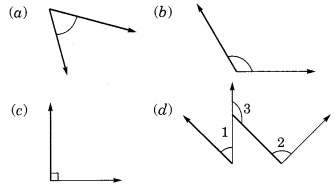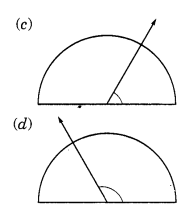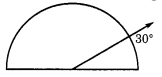## Ex 5.4 Question 1.

What is the measure of (i) a right angle (ii) a straight angle?
Solution:
(i) The measure of a right angle = 90°
(ii) The measure of a straight angle = 180°

## Ex 5.4 Question 2.

Say True or False:
(a) The measure of an acute angle < 90°
(b) The measure of an obtuse angle < 90°
(c) The measure of a reflex angle > 180°
(d) The measure of one complete revolution = 360°
(e) If m ∠A = 53° and ∠B = 35°, then m∠A > m∠B.
Solution:
(a) True
(b) False
(c) True
(d) True
(e) True

## Ex 5.4 Question 3.

Write down the measures of
(a) some acute angles
(b) some obtuse angles
Solution:
(a) 25°, 50°,65°, and 72° are acute angles.
(b) 105°, 120°, 135°, and 175° are obtuse angles.

## Ex 5.4 Question 4.

Measure the angles given below using the protractor and write down the measure.Solution:
(a) 45°
(b) 125°
(c) 90°
(d) ∠1 = 60°, ∠2 = 90°, ∠3 = 125°

## Ex 5.4 Question 5.

Which angle has a large measure? First, estimate and then measure.
The measure of Angle A =
The measure of Angle B =Solution:
The measure of Angle A = 40°
The measure of Angle B = 65°

## Ex 5.4 Question 6.

From these two angles which have large measures? Estimate and then confirm by measuring them.
Solution:∴ Measure of angle (a) = 45°
and the measure of angle (b) = 60°

The angle shown in the second figure is greater.

## Ex 5.4 Question 7.

Fill in the blanks with acute, obtuse, right or straight:
(a) An angle whose measure is less than that of a right angle is _____________.
(b) An angle whose measure is greater than that of a right angle is ___________ .
(c) An angle whose measure is the sum of the measures of two right angles is __________ .
(d) When the sum of the measures of two angles is that of a right angle, then each one of them is _________.
(e) When the sum of the measures of two angles is that of a straight angle and if one of them is acute then the other should be_________ .
Solution:
(a) acute
(b) obtuse
(c) straight
(d) acute
(e) obtuse

## Ex 5.4 Question 8.

Find the measure of the angle shown in each figure. (First estimate with your eyes and than find the actual measure with a protractor).Solution:
(a) The measure of the angle = 40°
(b) The measure of the angle = 130°
(c) The measure of the angle = 65°
(d) The measure of the angle = 135°.

## Ex 5.4 Question 9.

Find the angle measure between the hands of the clock in each figure:Solution:
(i) The angle between the hour hand and the minute hand of a clock at 9.00 a.m = 90° (Right angle)
(ii) The angle between the hour hand and minute hand of a clock at 1.00 p.m = 30° (Acute angle)
(iii) The angle between the hour hand and minute hand of a clock at 6.00 p.m = 180°. (Straight angle)

## Ex 5.4 Question 10.

Investigate: In the given figure, the angle measures 30°. Look at the same figure through a magnifying glass. Does the angle become larger? Does the size of the angle change?Solution:

The measure of an angle will be the same.

## Ex 5.4 Question 11.

Measure and classify each angle:Angle Measure Type
∠AOB
∠AOC
∠BOC
∠DOC
∠DOA
∠DOB
Angle Measure Type
∠AOB 40° Acute angle
∠AOC 125°Obtuse angle
∠BOC 85° Acute angle
∠DOC 95° Obtuse angle
∠DOA 140°Obtuse angle
∠DOB 180°Straight angle

error: Content is protected !!